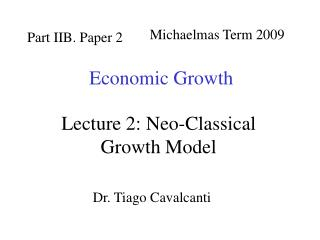DownloadDownload PresentationPart IIB. Paper 2

# Part IIB. Paper 2

Télécharger la présentation## Part IIB. Paper 2

- - - - - - - - - - - - - - - - - - - - - - - - - - - E N D - - - - - - - - - - - - - - - - - - - - - - - - - - -
##### Presentation Transcript

1. Michaelmas Term 2009 Part IIB. Paper 2 Economic Growth Lecture 2: Neo-Classical Growth Model Dr. Tiago Cavalcanti

2. Readings and Refs Texts: (*)Jones ch.2; BX chs.1,10; Romer ch.1. Original Articles: Solow R. (1956) ‘A contribution to the theory of economic growth’ Quarterly Journal of Economics, 70, 65-94. Solow R. (1957) ‘Technical change and the aggregate production function’ Review of Economics and Statistics, 39, 312-320. Swan T. (1956) ‘Economic growth and capital accumulation’ Economic Record, 32, 334-361.

3. The Neoclassical Growth modelSolow (1956) and Swan (1956) • simple dynamic general equilibrium model of growth

4. Neoclassical Production Function Output produced using aggregate production functionY = F (K , L ), satisfying: A1. positive, but diminishing returns FK >0, FKK<0and FL>0, FLL<0 A2. constant returns to scale (CRS) • replication argument

5. Production Function in Intensive Form • Under CRS, can write production function • Alternatively, can write in intensive form: • y = f ( k ) • - where per capitay = Y/L and k = K/L Exercise: Given that Y=L f(k), show: FK = f’(k) and FKK= f’’(k)/L .

6. Competitive Economy • representative firms maximise profits and take price as given (perfect competition) • can show: inputs paid their marginal products: r = FKand w = FL • inputs (factor payments) exhaust all output: wL + rK = Y • general property of CRS functions (Euler’s THM)

7. A3: The Production Function F(K,L) satisfies the Inada Conditions Note: As f’(k)=FKhave that Production Functions satisfying A1, A2 and A3 often called Neo-Classical Production Functions

8. Technological Progress = change in the production functionFt Hicks-Neutral T.P. Labour augmenting (Harrod-Neutral) T.P. Capital augmenting (Solow-Neutral) T.P.

9. A4: Technical progress is labour augmenting Note: For Cobb-Douglas case three forms of technical progress equivalent:

10. Under CRS, can rewrite production function in intensive form in terms of effective labour units • note: drop time subscript to for notational ease • Exercise: Show that

11. Model Dynamics A5: Labour force grows at a constant rate n A6: Dynamics of capital stock: • net investment = gross investment - depreciation • capital depreciates at constant rate 

12. … closing the model • National Income Identity • Y = C + I + G + NX • Assume no government (G = 0) and closed economy (NX = 0) • Simplifying assumption: households save constant fraction of income with savings rate 0  s  1 • I = S = sY • Substitute in equation of motion of capital:

13. Fundamental Equation of Solow-Swan model

14. Steady State Definition: Variables of interest grow at constant rate (balanced growth pathor BGP) • at steady state:

15. Solow Diagram

16. Existence of Steady State • From previous diagram, existence of a (non-zero) steady state can only be guaranteed for all values of n,g and d if - satisfied from Inada Conditions (A3).

17. Transitional Dynamics • If , then savings/investment exceeds “depreciation”, thus • If , then savings/investment lower than “depreciation”, thus • By continuity, concavity, and given that f(k) satisfies the INADA conditions, there must exists an unique

18. Transitional Dynamics

19. Properties of Steady State 1. In steady state, per capita variables grow at the rate g, and aggregate variables grow at rate(g + n) Proof:

20. 2. Changes in s, n, or dwill affect the levels of y* and k*, but not the growth rates of these variables. - Specifically, y* and k* will increase as s increases, and decrease as either n or dincrease Prediction: In Steady State, GDP per worker will be higher in countries where the rate of investment is high and where the population growth rate is low - but neither factor should explain differences in the growth rate of GDP per worker.

21. Golden Rule and Dynamic Inefficiency • Definition: (Golden Rule) It is the saving rate that maximises consumption in the steady-state. • Given we can use to find .

22. Golden Rule and Dynamic Inefficiency

23. Changes in the savings rate • Suppose that initially the economy is in the steady state: • If s increases, then • Capital stock per efficiency unit of labour grows until it reaches a new steady-state • Along the transition growth in output per capita is higher than g.

24. Linear versus log scales

25. Changes in the savings rate

26. Next lecture Testing the neo-classical model: • Convergence • Growth Regressions • Evidence from factor prices